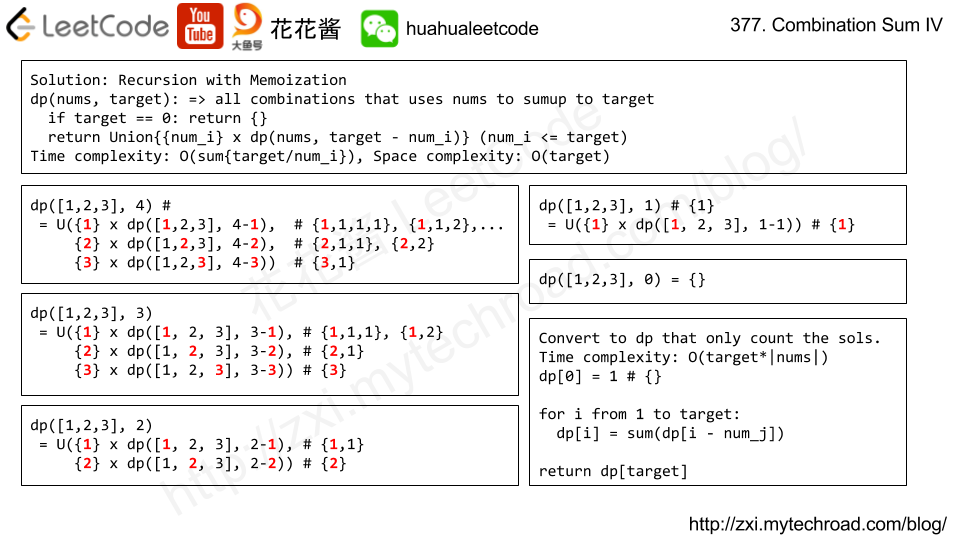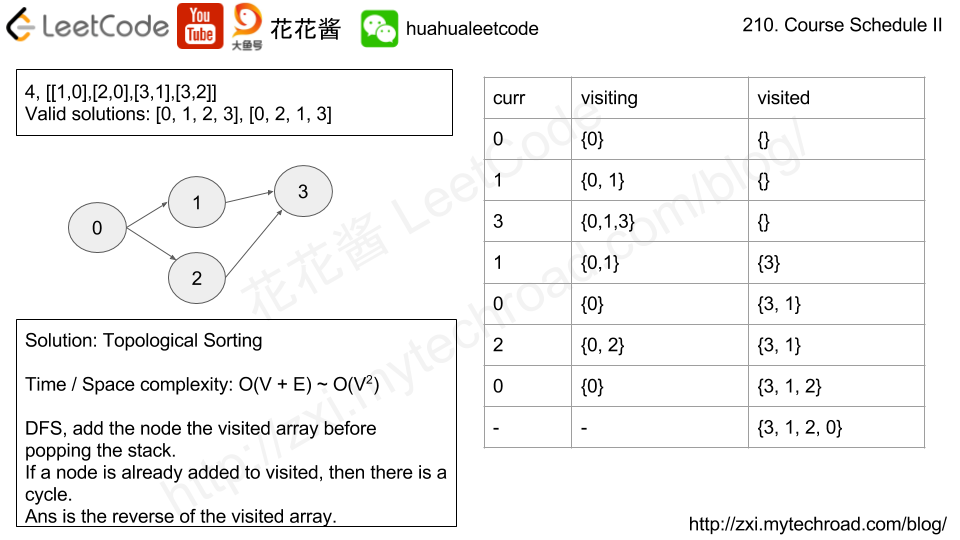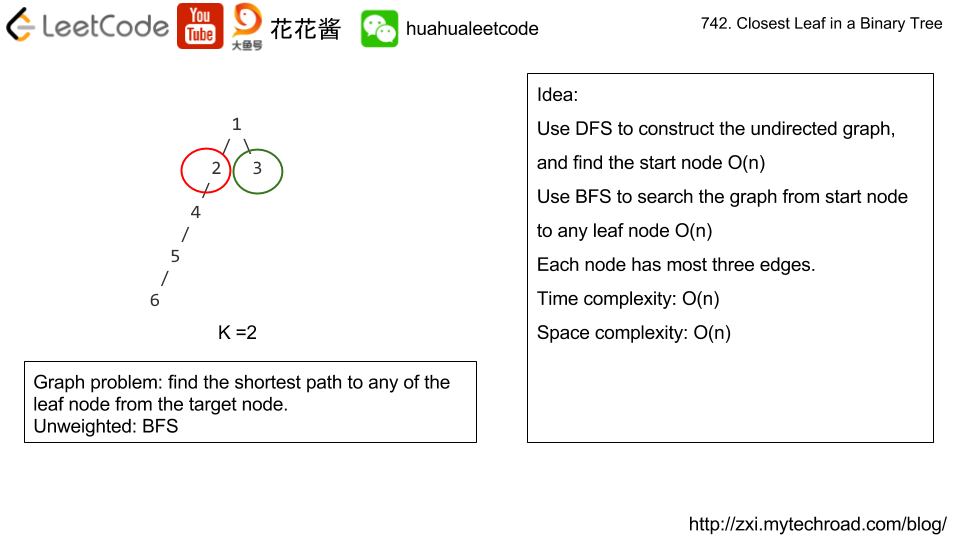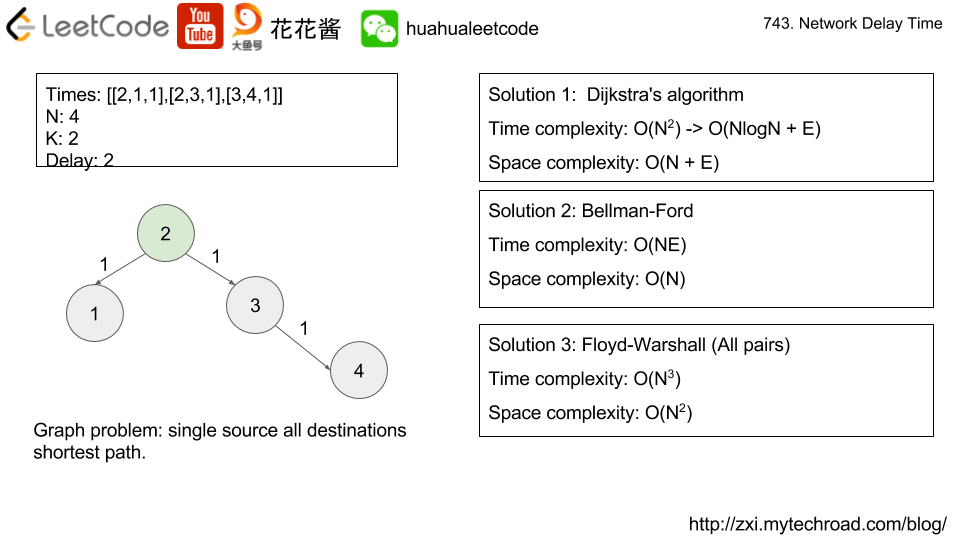# Posts published in “Medium”

Problem:

Given a non-empty array containing only positive integers, find if the array can be partitioned into two subsets such that the sum of elements in both subsets is equal.

Note:

1. Each of the array element will not exceed 100.
2. The array size will not exceed 200.

Example 1:

Example 2:

Idea:

DP 动态规划

Solution:

Time complexity: O(n*sum)

Space complexity: O(sum)

C++

Problem:

Given an integer array with all positive numbers and no duplicates, find the number of possible combinations that add up to a positive integer target.

Example:

Idea:

DPSolution:

C++ / Recursion + Memorization

C++ / DP

Related Problems:

# Problem

There are a total of n courses you have to take, labeled from 0 to n - 1.

Some courses may have prerequisites, for example to take course 0 you have to first take course 1, which is expressed as a pair: [0,1]

Given the total number of courses and a list of prerequisite pairs, return the ordering of courses you should take to finish all courses.

There may be multiple correct orders, you just need to return one of them. If it is impossible to finish all courses, return an empty array.

For example:

There are a total of 2 courses to take. To take course 1 you should have finished course 0. So the correct course order is [0,1]

There are a total of 4 courses to take. To take course 3 you should have finished both courses 1 and 2. Both courses 1 and 2 should be taken after you finished course 0. So one correct course order is [0,1,2,3]. Another correct ordering is[0,2,1,3].

Note:

1. The input prerequisites is a graph represented by a list of edges, not adjacency matrices. Read more about how a graph is represented.
2. You may assume that there are no duplicate edges in the input prerequisites.# Idea

Topological sorting

# Solution 1: Topological Sorting

Time complexity: O(V+E)

Space complexity: O(V+E)

# Related Problems:

Problem:

Given a binary tree where every node has a unique value, and a target key k, find the value of the closest leaf node to target k in the tree.

Here, closest to a leaf means the least number of edges travelled on the binary tree to reach any leaf of the tree. Also, a node is called a leaf if it has no children.

In the following examples, the input tree is represented in flattened form row by row. The actual root tree given will be a TreeNode object.

Example 1:

Example 2:

Example 3:

Note:

1. root represents a binary tree with at least 1 node and at most 1000 nodes.
2. Every node has a unique node.val in range [1, 1000].
3. There exists some node in the given binary tree for which node.val == k.

Idea:

Shortest path from source to any leaf nodes in a undirected unweighted graph.Solution:

C++ / DFS + BFS

Time complexity: O(n)

Space complexity: O(n)

There are N network nodes, labelled 1 to N.

Given times, a list of travel times as directed edges times[i] = (u, v, w), where u is the source node, v is the target node, and w is the time it takes for a signal to travel from source to target.

Now, we send a signal from a certain node K. How long will it take for all nodes to receive the signal? If it is impossible, return -1.

Note:

1. N will be in the range [1, 100].
2. K will be in the range [1, N].
3. The length of times will be in the range [1, 6000].
4. All edges times[i] = (u, v, w) will have 1 <= u, v <= N and 1 <= w <= 100.

Idea:

Construct the graph and do a shortest path from K to all other nodes.Solution 2:

C++ / Bellman-Ford

Time complexity: O(ne)

Space complexity: O(n)

Solution3:

C++ / Floyd-Warshall

Time complexity: O(n^3)

Space complexity: O(n^2)

v2

Mission News Theme by Compete Themes.